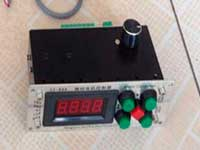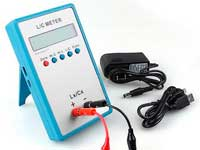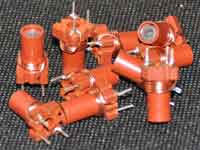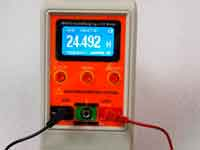Ferrite toroid calculation - 4.4 out of 5 based on 7 votes
Rating 4.43 (7 Votes)

Ferrite toroid calculationToroidal coils on ferrite ring or rings of carbonyl iron powder are widely used in amateur radio designs. Their advantage is the high combined inductance with the small stray field. Calculation of the coil can be carried out in various ways. The most used method for calculation is based on a special parameter AL. This parameter is usually included in the specification of the ferromagnetic rings and published by manufacturers [Example for the Amidon™ ferrite ring]. Numerical parameter AL is the inductance in uH at 100 turns of the coil for the iron powder ring or the inductance in mH at 1000 turns of the coil for the ferrite ring. Knowing the AL parameter, the number of windings of the toroid can be calculated using the following expressions:The first formula for the iron powder ring, the second formula for the ferrite ring.

The calculation in the Coil32 program is based on two empirical formulas. For the calculation by these formulas is enough to know the dimensions of the ring and its permeability.at D1/D2>1.75at D1/D2<1.75

All dimensions are in millimeters, the inductance in uH. μ is the relative magnetic permeability of the core. In the case of low-current inductors, you can assume it as initial magnetic permeability. These formulas are not applicable for power chokes calculation.
At the same time, by the numerical algorithm, the program calculates the length of wire needed for the winding. Wire length is calculated with a margin of 10 cm on the "ends". If on finish you see string:"Coil cannot be realized!", it means that the wire cannot be placed in the inner diameter of the ferromagnetic ring and you must decrease the diameter of a wire. Calculation of inductance on a ferrite ring in the online calculator is based on the same formulas.

The program includes plugin named Ring_permeability, which allows you to calculate an unknown permeability of ferrite ring. Let's say you have a ferromagnetic ring, and you know nothing about its characteristics. In this case, it is necessary to wind a few turns on the ring and then to measure the resulting inductance and the dimensions of the ring. From these data, the plugin Ring_permeability allows estimating permeability of the ring. The calculation uses the same empirical formulas.

Сomments from anonymous guests are enabled with moderation.

Coil Winding MachineUS \$187.53
The system consists of individual components including a controller, a stepping motor, a foot switch, power adapter and output shaft. This design makes it very easy for you to integrate the system into your own project.

LC Meter LC200AUS \$37.99
Measuring Capacitance Range:
0.01 pF - 100mF
Measuring Inductance Range:
0.001 uH - 100H
Measurement accuracy:1%.

RF InductorsUS \$6.43
Variable Ferrite Core Inductor coil set 3.5Turns 25-100MHZ

Auto Ranging LCR MeterUS \$63.07
Meter can measure 0.00pF to 100.00mF capacitance, 0.000uH to 100.00H inductance and 0.00? to 10.000M? resistance. Very suitable for small value capacitor and inductor measurement.